Open in App
Not now

# Class 11 RD Sharma Solutions – Chapter 15 Linear Inequations – Exercise 15.5

• Difficulty Level : Medium
• Last Updated : 13 Jan, 2021

### Question 1. x+2y-4 <=0

Solution:

Step 1. Convert the given inequation into equation i.e. x+2y-4 =0

Step 2. Plot the obtained equation on cartesian plane.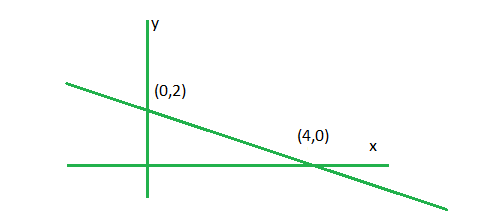Step 3. Take two points lying on each side of line and put it in inequality, the one which holds the inequality true ,the required plot will be towards that point.

Here we take (0,0) and (5,0).

Putting these points in x+2y-4 <= 0. (5,0) satisfies.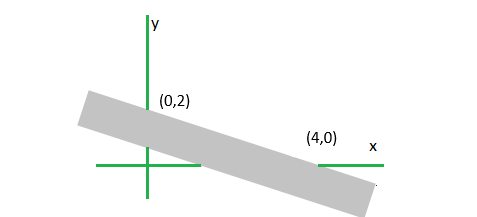### Question 2. x+2y >= 6

Solution:

First writing the equation as x+2y-6 >=0.

Step 1. Convert the given inequation into equation i.e. x+2y-6 = 0.

Step 2. Plot the obtained equation on Cartesian plane.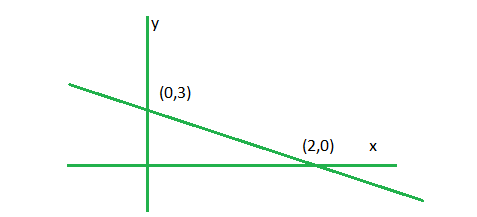Step 3. Take two points lying on each side of line and put it in inequality, the one which holds the inequality true ,the required plot will be towards that point .

Here we take (0,0) and (3,0).

Putting these points in x+2y-6 >=0. (3,0) satisfies.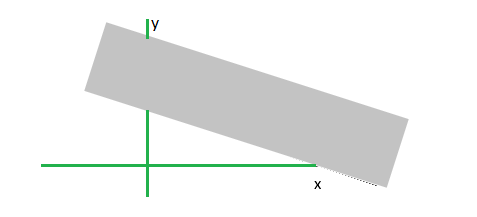### Question 3. x+2 >= 0.

Solution:

Step 1. Convert the given inequation into equation i.e. x+2 = 0

Step 2. Plot the obtained equation on cartesian plane.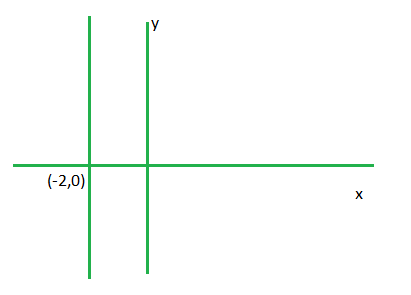Step 3. Here inequality have one variable so x >= -2 ,hence shade the obtained region i.e.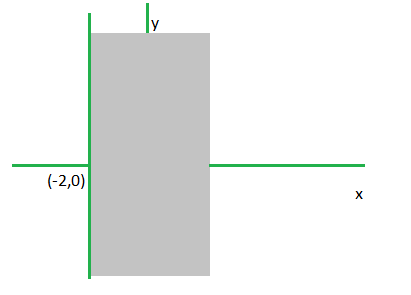### Question 4. x-2y < 0.

Solution:

Step 1. Convert the given inequation into equation i.e. x – 2y = 0

Step 2. Plot the obtained equation on cartesian plane.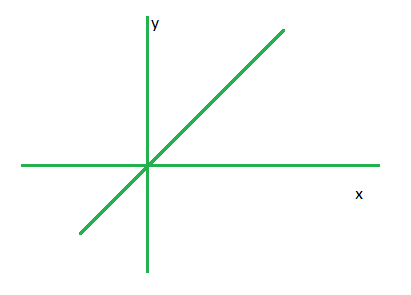Step 3. Take two points lying on each side of line and put it in inequality, the one which holds the inequality true ,the required plot will be towards that point

Here we take (0, 2) and (2, 0)

Putting these points in x-2y<0, (0, 2) satisfies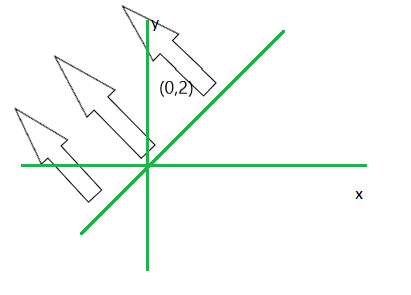### Question 5. -3x+2y <= 6

Solution:

Firstly writing the inequation as -3x+2y-6 <= 0.

Step 1. Convert the given inequation into equation i.e. -3x+2y-6 = 0.

Step 2. Plot the obtained equation on cartesian plane.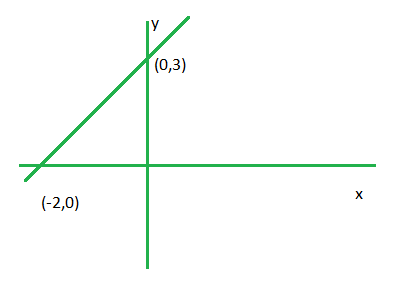Step 3. Take two points lying on each side of line and put it in inequality, the one which holds the inequality true ,the required plot will be towards that point.

Here we take (-1,0) and (-3,0).

Putting these points in -3x+2y-6 <= 0. (-1, 0) satisfies.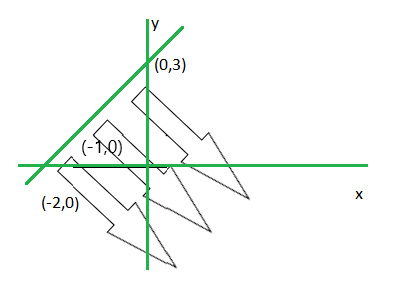### Question 6. x <= 8-4y.

Solution:

At first write the inequality as x+4y-8 <= 0.

Step 1. Convert the given inequation into equation i.e. x+4y-8 = 0

Step 2. Plot the obtained equation on cartesian plane.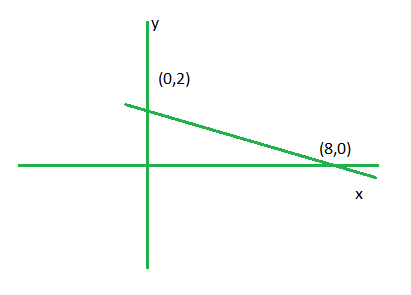Step 3. Take two points lying on each side of line and put it in inequality, the one which holds the inequality true ,the required plot will be towards that point

Here we take (0,0) and (9,0).

Putting these points in x+4y-8 <= 0. (0, 0) satisfies.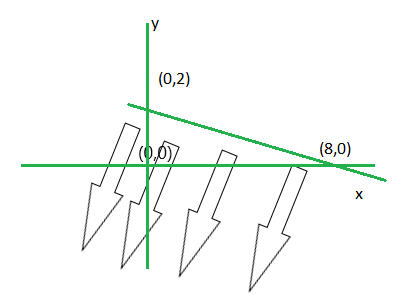### Question 7. 0 <= 2x-5y+10

Solution:

First read the inequality as 2x-5y+10 >= 0.

Step 1. Convert the given inequation into equation i.e. 2x-5y+10 = 0

Step 2. Plot the obtained equation on cartesian plane.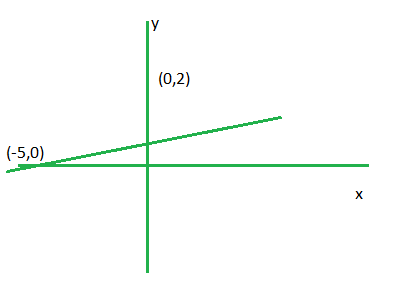Step 3. Take two points lying on each side of line and put it in inequality, the one which holds the inequality true ,the required plot will be towards that point.

Here we take (0,0) and (-6,0)

Putting these points in 2x-5y+10 >= 0. (0,0) satisfies.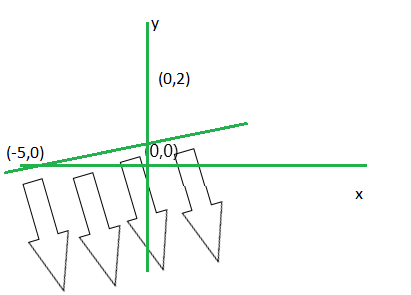### Question 8. 3y > 6-2x

Solution:

First manipulate the inequation as 2x+3y-6 >0.

Step 1. Convert the given inequation into equation i.e.2x+3y-6= 0

Step 2. Plot the obtained equation on cartesian plane.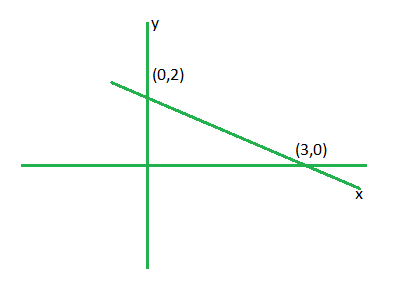Step 3. Take two points lying on each side of line and put it in inequality, the one which holds the inequality true ,the required plot will be towards that point.

Here we take (0,0) and (4,0)

Putting these points in 2x+3y-6 >0 (4,0) satisfies.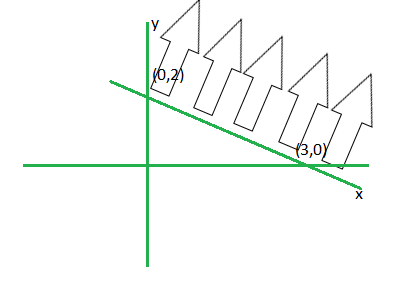### Question 9. y > 2x-8

Solution:

First convert the inequation as -2x+y+8 >0.

Step 1. Convert the given inequation into equation i.e. -2x+y+8= 0

Step 2. Plot the obtained equation on cartesian plane.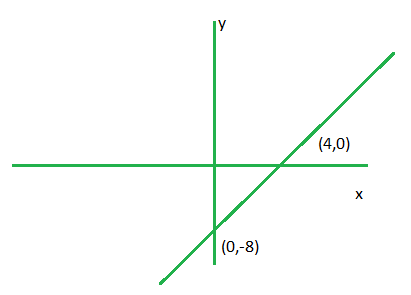Step 3. Take two points lying on each side of line and put it in inequality, the one which holds the inequality true, the required plot will be towards that point.

Here we take (0,0) and (5,0)

Putting these points in -2x+y+8 >0 (0,0) satisfies.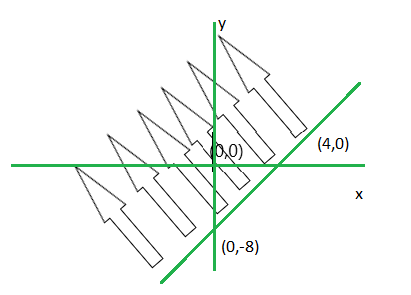### Question 10. 3x-2y <= x+y-8

Solution:

First take all the variables in one side i.e. 2x-3y+8 <= 0.

Step 1. Convert the given inequation into equation i.e. 2x-3y+8 = 0

Step 2. Plot the obtained equation on cartesian plane.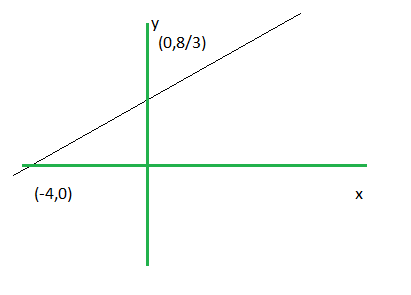Step 3. Take two points lying on each side of line and put it in inequality, the one which holds the inequality true ,the required plot will be towards that point.

Here we take (0,0) and (-5,0)

Putting these points in 2x-3y+8 <= 0. (-5,0) satisfies.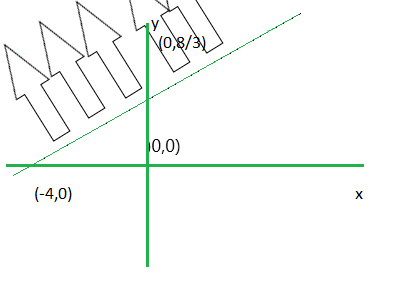My Personal Notes arrow_drop_up